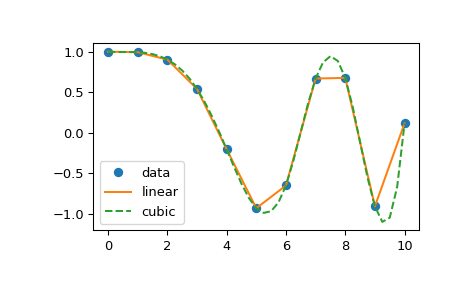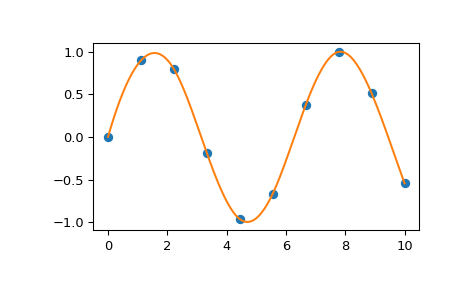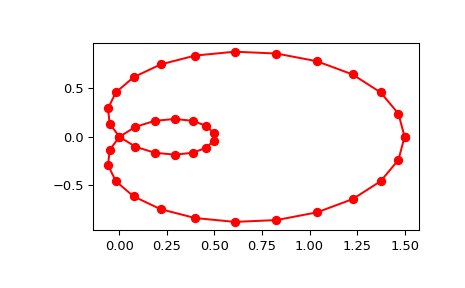Scipy 学习 第1篇：插补

scipy包提供了几种通用的插补工具，分别用于1维，2维或高维的数据集，分别是：

• 类 interp1d 用于对一维数据进行插补
• 类 griddata 提供了对1D，2D和高维数据进行插补的工具。
• spline插补， 用于对一维和二维数据进行平滑的cubic-spline 插补
• 使用基于径向的函数（RBF）进行插值

一，interp1d类

scipy.interpolate.interp1d(x, y, kind='linear', axis=-1, copy=True, bounds_error=None, fill_value=nan, assume_sorted=False)

kind 参数用于指定内推的类型，(‘linear’, ‘zero’, ‘slinear’, ‘quadratic’, ‘cubic’, ‘previous’, ‘next’和 ‘nearest’。对于'linear'，是默认值，表示线性插补，前面已描述；对于 ‘zero’, ‘slinear’, ‘quadratic’ 和 ‘cubic’ 引用的是spline内推，使用 zeroth, first, second 和 third 顺序。对于 ‘previous’ 、 ‘next’ 和  ‘nearest’ 简单返回数据点的前一个、后一个或最近的一个数据。

>>> import scipy
>>> from scipy.interpolate import interp1d
>>> x = np.linspace(0, 10, num=11, endpoint=True)
>>> y = np.cos(-x**2/9.0)
>>> f = interp1d(x, y)
>>> f2 = interp1d(x, y, kind='cubic')
>>> xnew = np.linspace(0, 10, num=41, endpoint=True)
>>> import matplotlib.pyplot as plt
>>> plt.plot(x, y, 'o', xnew, f(xnew), '-', xnew, f2(xnew), '--')>>> plt.legend(['data', 'linear', 'cubic'], loc='best')
>>> plt.show()二，多变量插补（griddata）

scipy.interpolate.griddata(points, values, xi, method='linear', fill_value=nan, rescale=False)

• points：自变量，对于二元函数而言，points是指由(x,y)构成的数据点
• values：因变量，values是指某一个数据点的值，对于二元函数而言，数据点(x,y)的值是z=f(x,y)
• xi：坐标系，或者说是数据点在坐标系中的位置，也可以把points的值作为坐标系的x和y轴，把values作为坐标系的z轴
• method：有效值是‘linear’, ‘nearest’, ‘cubic’

三，spline类

1，直接方式

splrep()函数的作用是对于给定数据点集（x [i]，y [i]），确定在间隔xb <= x <= xe上平滑度为k的近似值。

scipy.interpolate.splrep(x, y, w=None, xb=None, xe=None, k=3, task=0, s=None, t=None, full_output=0, per=0, quiet=1)

• x和y定义一个曲线，y=f(x)
• w 权重，用于在spline拟合时计算加权最小二乘法的值，w的默认值为1/(len(x))。如果y值中的误差是向量d给出的标准偏差，则w应为1 / d。
• xb，xe：用于拟合的间隔，如果没有指定，那么默认值分别是x 和 x[-1]。
• k  用于指定spline order，默认值是3，字符串表示为cubic，k的取值区间是[1,5]
• s 用于制定平滑条件，满足以下条件来确定平滑度：sum（（w *（y-g））** 2，axis = 0）<= s，其中g（x）是（x，y）的平滑插值。 用户可以使用s来控制贴合度和平滑度之间的权衡，较大的s表示更平滑，而较小的s值表示较不平滑。s的推荐值取决于权重w。 如果权重代表y的标准偏差的倒数，则应在（m-sqrt（2 * m），m + sqrt（2 * m））范围内找到一个好的s值，其中m是 x，y和w中的数据点。 默认值：如果提供了权重，则s = m-sqrt（2 * m）。 如果未提供权重，则s = 0.0（内插）。

scipy.interpolate.splev(x, tck, der=0, ext=0)

>>> import matplotlib.pyplot as plt
>>> from scipy.interpolate import splev, splrep
>>> x = np.linspace(0, 10, 10)
>>> y = np.sin(x)
>>> spl = splrep(x, y)
>>> x2 = np.linspace(0, 10, 200)
>>> y2 = splev(x2, spl)
>>> plt.plot(x, y, 'o', x2, y2)
>>> plt.show()2，参数化方式

scipy.interpolate.splprep(x, w=None, u=None, ub=None, ue=None, k=3, task=0, s=None, t=None, full_output=0, nest=None, per=0)

• x：代表N维空间中曲线的N个数组的列表，每个数组的长度都是曲线的点的数量，每一个数组都提供N维数据点的一个分量。
• u：是参数值的数组，该参数默认为0到1之间的等距单调序列，计算公式是：

M = len(x), where v = 0
v[i] = v[i-1] + distance(x[i], x[i-1])
u[i] = v[i] / v[M-1]

• ub,ue：参数间隔的端点，默认值是 u 和u [-1]。
• k：spline拟合的度，推荐使用默认值k=3，表示cubic，k的取值区间是[1,5]
• s：平滑度，是0到1之间的小数，0表示不平滑，值越大，平滑度越高。
• per：如果非0，那么把数据点视为周期性曲线，周期为x [m-1]-x ，并返回平滑的周期样条近似。

>>> from scipy.interpolate import splprep, splev
>>> tck, u = splprep([x, y], s=0)
>>> new_points = splev(u, tck)
>>> import matplotlib.pyplot as plt
>>> fig, ax = plt.subplots()
>>> ax.plot(x, y, 'ro')
>>> ax.plot(new_points, new_points, 'r-')
>>> plt.show()SciPy documentation

posted @ 2018-08-14 10:32  悦光阴  阅读(1647)  评论(0编辑  收藏  举报